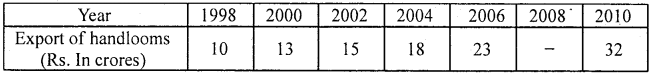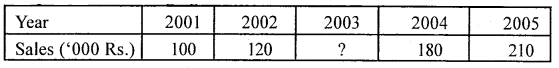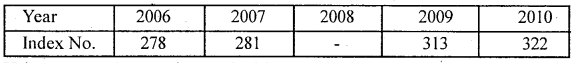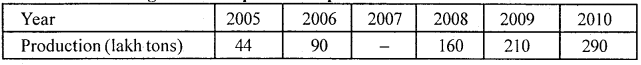# 1st PUC Statistics Question Bank Chapter 8 Interpolation and Extrapolation

## Karnataka 1st PUC Statistics Question Bank Chapter 8 Interpolation and Extrapolation

### 1st PUC Interpolation and Extrapolation Question and Answers

Question 1.
What is Interpolation?
‘Interpolation is a procedure of estimating the unknown value of dependent variable for a given value of independent variable which is within the limits or the range of the independent variable’

Question 2.
What is extrapolation?
Extrapolation is a procedure of estimating the unknown value of dependent variable for a given value of independent variable which is outside the limits or the range of the independent variable’

Question 3.
Mention the situations where the technique of interpolation is used.
The procedure of estimating the missing value of y for a given value of x, where x is within the limits x0 and xn we use the technique Interpolation.

Question 4.
What are the assumptions made in interpolation?
In making use of the techniques of interpolation the following assumptions are made

• There are no sudden jumps in the values of independent variable from one period to another.
• The rate of change of figures from one period to another is uniform.Question 5.
Using Binomial expansion method expand (y – 1)5 = 0.
The equation (y – 1 )5 = 0 is:
y5 – 5y-4 + 10y3 -10y2 + 5y1 -y0 = 0

Question 6.
If in a Binomial expansion y4 – 4y3 + 6y2 – 4y1 + y0 = 0, if y3=157, y2=124, y1 = 107 and  y0=100, then find y4
Substituting the known values in the equation, we obtain,
y4 – 4(157) + 6(124) – 4(107)+ 100 = 0
y4 – 1056 + 844= 0
y4 – 212= 0
∴ y4 = 212

Question 7.
Distinguish between interpolation and extrapolation.
The procedure of estimating the missing value of y for a given value of x, where x is within the limits x0 and xn we use Interpolation. Here “Interpolation is a procedure of estimating the unknown value of dependent variable for a given value of independent variable which is within the limits or the range of the independent variable”

But if the value of y is to be estimated for a value of x which is outside the limits x0 and xn then procedure Extrapolation is used. “Extrapolation is a procedure of estimating the unknown value of dependent variable for a given value of independent variable which is outside the limits or the range of the independent variable”

Question 8.
Interpolate the export of handlooms during 2008 from the following dataLet X and Y be years and Exports

 (years)X (Export in crores Rs. ) Y 1998 (x0) 10 (y0) 2000 (x1) 13 (y1) 2002 (x2) 15 (y2) 2004 (x3) 23 (y3) 18 2006 (x4) 26 (y4) 23 2008 (x5) – (y5) 2010 (x6) 32 (y6)

Using binomial expansion method: Here we have to estimate y5 Since there are 6 known values of y, the formula of estimation is based on the expansion of (y-1)6= 0
We get the equation,
y6 – 6y5 + 15y4 – 20y3 + 15y2 – 6y1 + y0= 0
Substituting the known values in the equation, we get,
i.e.32 – 6y5+ 15 x 23 -20 x 18 + 15 x15 -6 x 13 + 10 = 0
i.e.32 – 6y5+ 345 – 360 + 225 – 78 + 10 = 0 ;
-6y5 = -174
⇒ y5 = 29
Hence the probable export for the year 2008 is 29 (crores Rs.)Question 9.
Interpolate the missing figure.Let X and Y be years and sales.

 (years)X (sales ) Y 2001 (x0) 100 (y0) 2002 (x1) 120 (y1) 2003 (x2) (y2) 2004 (x3) 180 (y3) 2005 (x4) 210 (y4)

Here we have to estimate y2 Since there are 4 known values of y, the formula of estimation is based on the expansion of (y – 1)4= 0
We get the equation,
Y4 -4 y3 + 6y2 – 4y1 + y0=o
Substituting the known values in the equation, we obtain,
i.e.210 – 4 x 180 + 6y2 – 4 x 120 +100 = 0
i.e.210 – 720 + 6y2 – 480 +100 = 0;
6y5= 890
⇒ y2 = 148.33
Hence the probable sales for the 2003 is 148.33

Question 10.
Interpolate the index for 2008 from the following data.Using Binomial expansion method – let X and Y be year and Index number.

 (Years) x (Index No.) y 2006 (x0) 278 (y0) 2007 (x1) 281 (y1) 2008 (x2) – (y2) 2009 (x3) 313 (y3) 2010 (x4) 322 (y4)

We have to estimate y2 Since there are 4 known values of y, the formula of estimation is • based on the expansion of (y – 1)4=0
We get the equation,
y4 – 4y3 + 6y2 – 4y1 yo = 0
Substituting the known values in the equation, we get,
i.e. 322 – 4(313) + 6y2 – 4(281) + 278 = 0
i.e. 322 – 1252 + 6y2 – 1124 + 278 = 0
6y2= 1776
⇒ y2 = 296
Hence the probable value of the index number of 2008 is 296.

Question 11.
From the following data interpolate the production of cement in 2007.Let X and Y be the year and production

 X Y 2005 (x0) 44 (y0) 2006 (x1) 90 (y1) 2007 (x2) (y2) 2008 (x3) 160 (y3) 2009 (x4) 210 (y4) 2010 (x5) 290 (y5)

Using Binomial expansion method We have to estimate y2 Since there are 5 known values of y, the formula of estimation is, the expansion of (y-1)5 = 0 We get the equation,
Y5 – 5y4 + 10y3 – 10y2 + 5y1 – y0= 0.
Substituting the known values in the equation we obtain,
290 – 5(210)+ 10 x 160 – 10y2+ 5(90) – 44 =0
290 – 1050+ 1600 – 10y2 + 450 – 44 =0
-10y2 – 1246 =0
– 10y2 =1246
∴ y2 = $$\frac { 1246 }{ 10 }$$
⇒ y2 = 127 is 124.6.